Gravitational Field Gravitational Potential Energy AP Physics C

• Slides: 22-Gravitational Field -Gravitational Potential Energy AP Physics C Mrs. CoyleRemember: Newton’s Law of Universal Gravitation -Universal Gravitation Constant G=6. 67 x 1011 Nm 2/kg 2 -The gravitational force is a field force.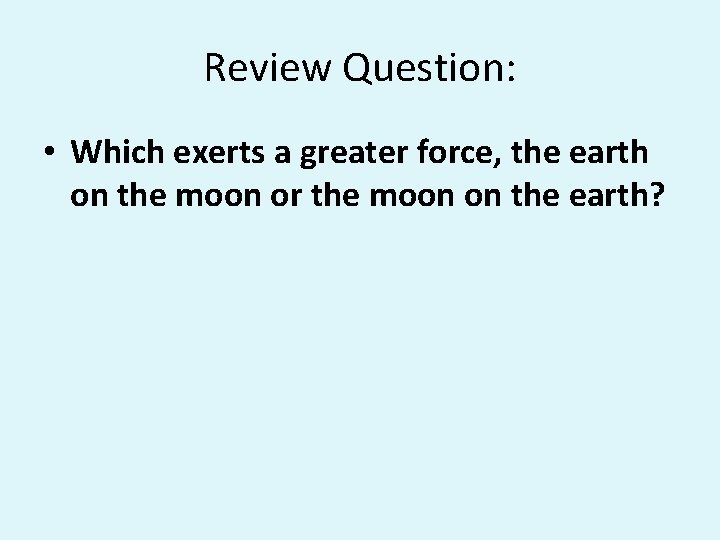Review Question: Question • Which exerts a greater force, the earth on the moon or the moon on the earth?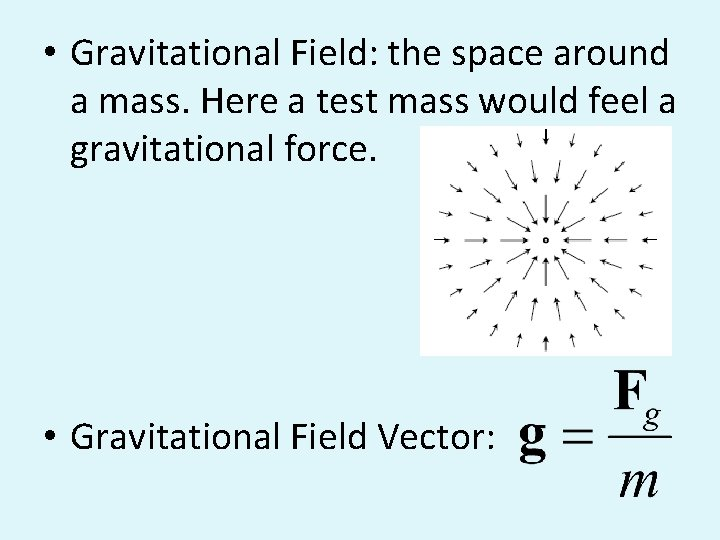• Gravitational Field: the t space around a mass. Here a test mass would feel a gravitational force. • Gravitational Field Vector: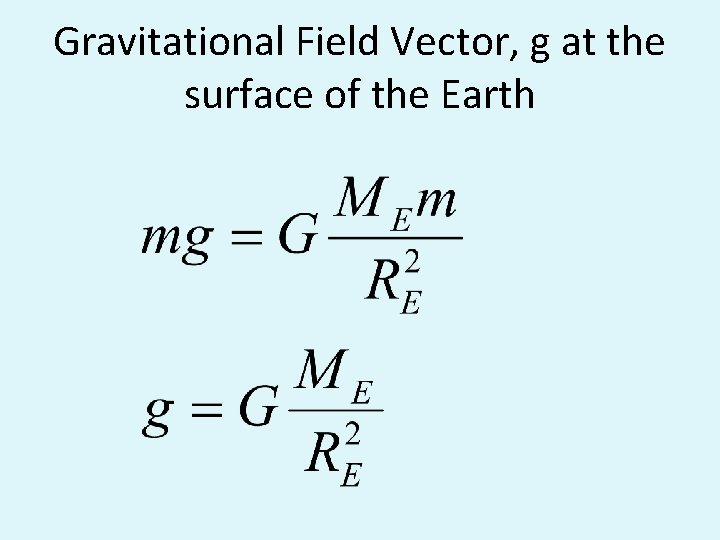Gravitational Field Vector, g at the surface of the Earth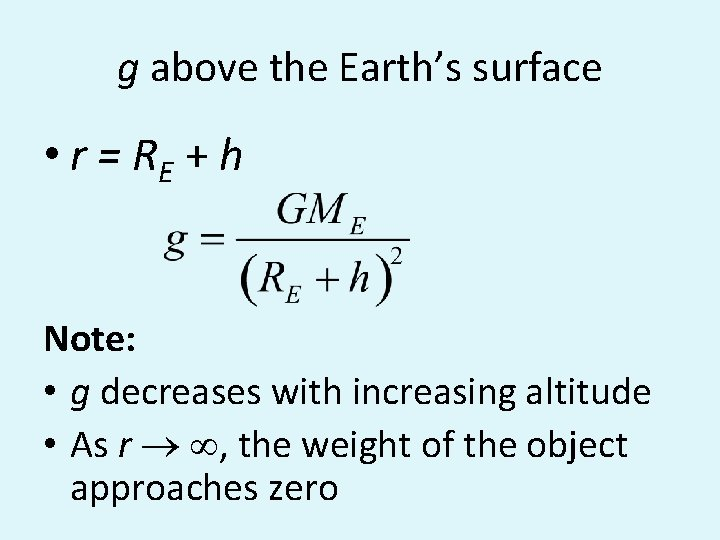g above the Earth’s surface • r = RE + h Note: • g decreases with increasing altitude • As r ® , the weight of the object approaches zero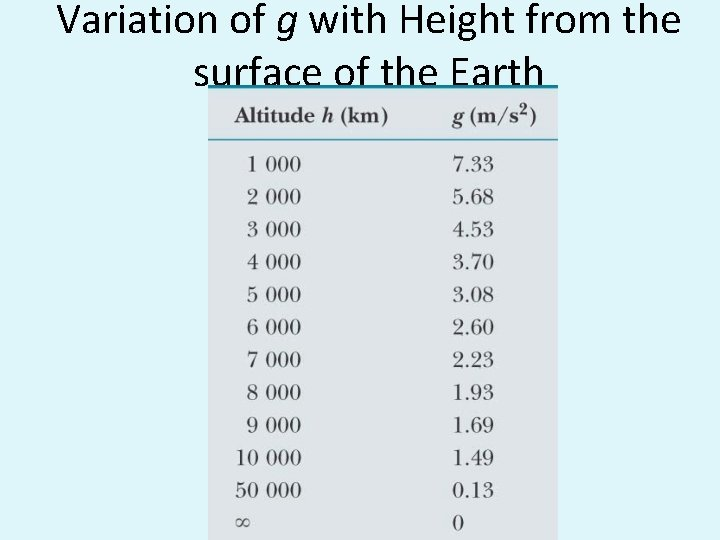Variation of g with Height from the surface of the Earth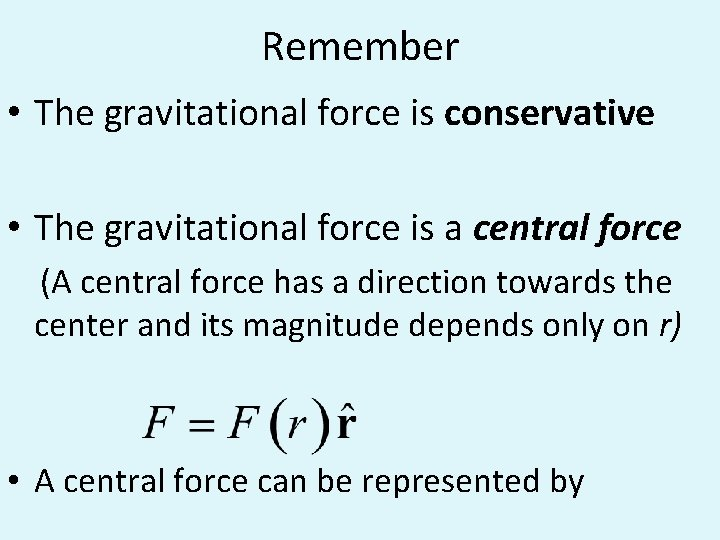Remember • The gravitational force is conservative • The gravitational force is a central force (A central force has a direction towards the center and its magnitude depends only on r) • A central force can be represented byWork done by the Gravitational Force • A particle moves from A to B while acted on by a central force F • We approximate the path along A to B with radial and arc zigzags • The work done by F along the arcs is zero • The work done by F along the radial direction is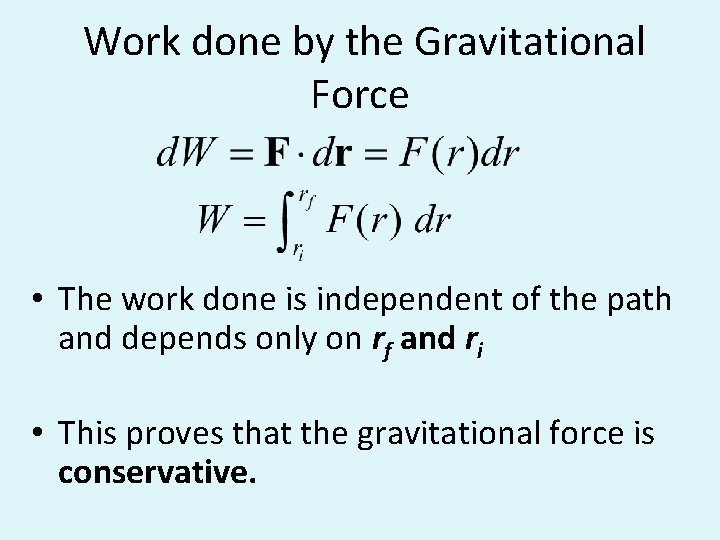Work done by the Gravitational Force • The work done is independent of the path and depends only on rf and ri • This proves that the gravitational force is conservative.Gravitational Potential Energy • As a particle moves from A to B, its gravitational potential energy changes by: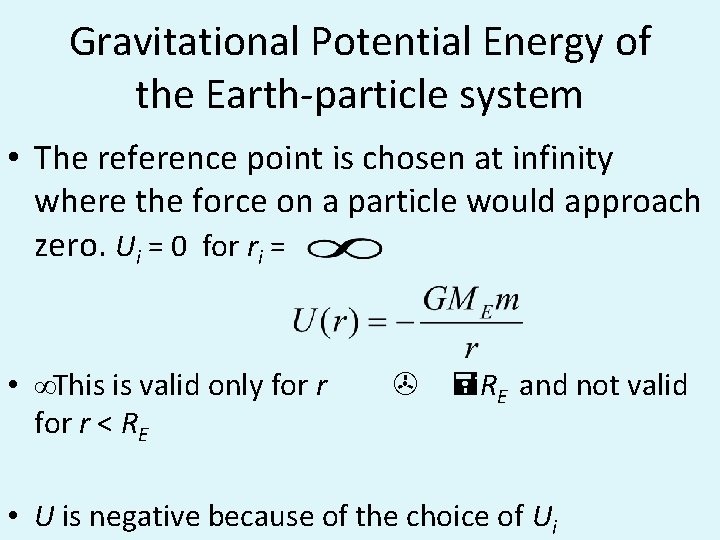Gravitational Potential Energy of the Earth-particle system • The reference point is chosen at infinity where the force on a particle would approach zero. Ui = 0 for ri = • ∞This is valid only for r < RE > =RE and not valid • U is negative because of the choice of UiGravitational Potential Energy of the Earthparticle system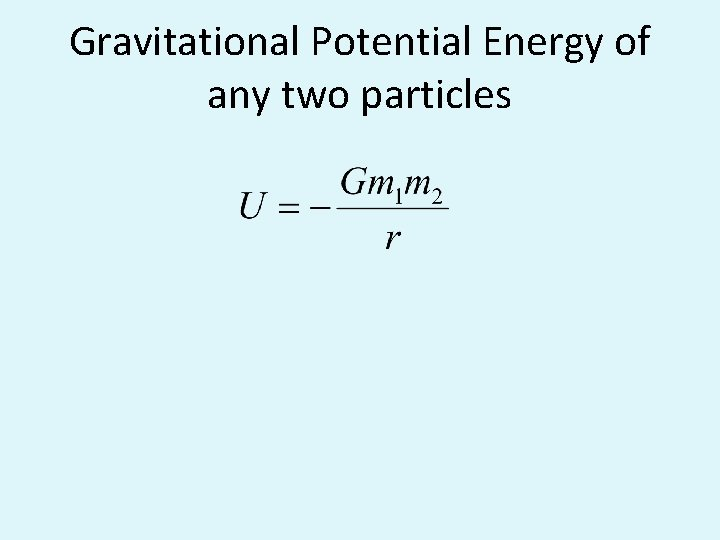Gravitational Potential Energy of any two particlesGravitational Potential Energy of a system of any two particles • U = -Gm 1 m 2 r The reference point U=0 is at infinity.Gravitational Potential Energy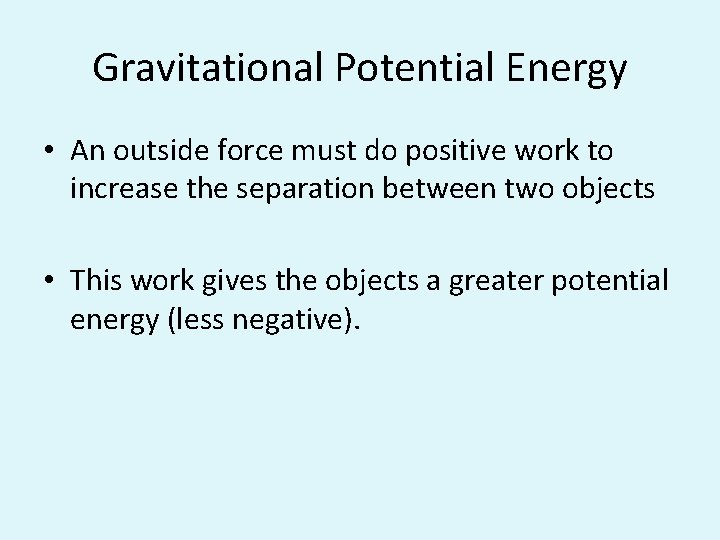Gravitational Potential Energy • An outside force must do positive work to increase the separation between two objects • This work gives the objects a greater potential energy (less negative).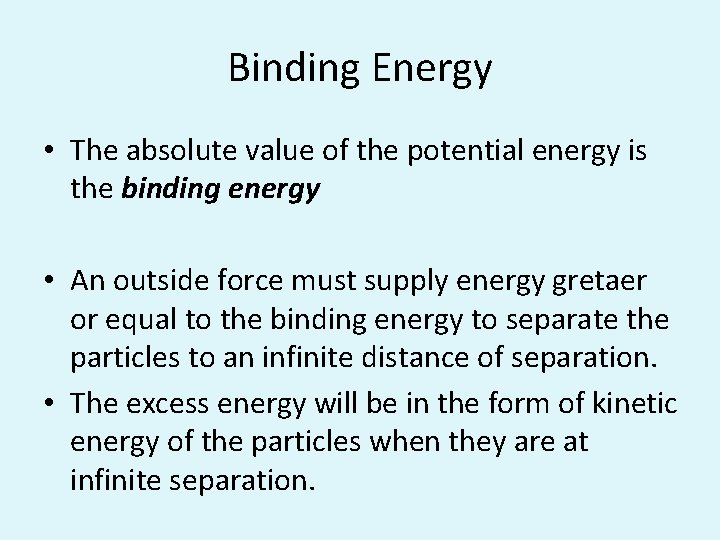Binding Energy • The absolute value of the potential energy is the binding energy • An outside force must supply energy gretaer or equal to the binding energy to separate the particles to an infinite distance of separation. • The excess energy will be in the form of kinetic energy of the particles when they are at infinite separation.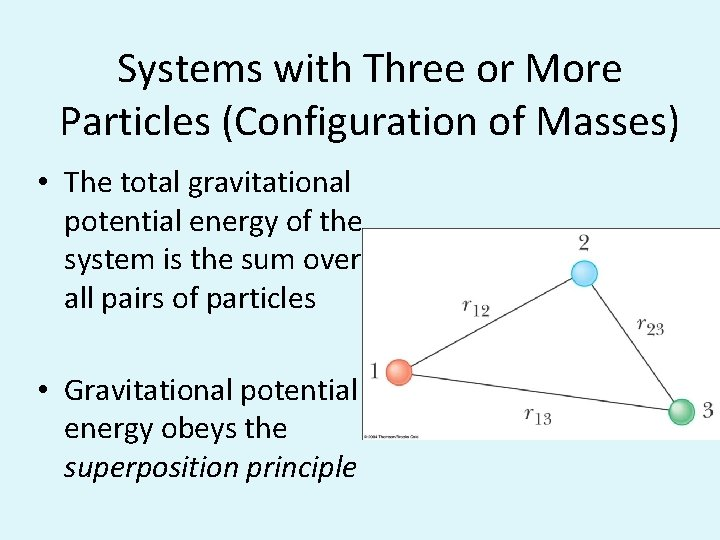Systems with Three or More Particles (Configuration of Masses) • The total gravitational potential energy of the system is the sum over all pairs of particles • Gravitational potential energy obeys the superposition principle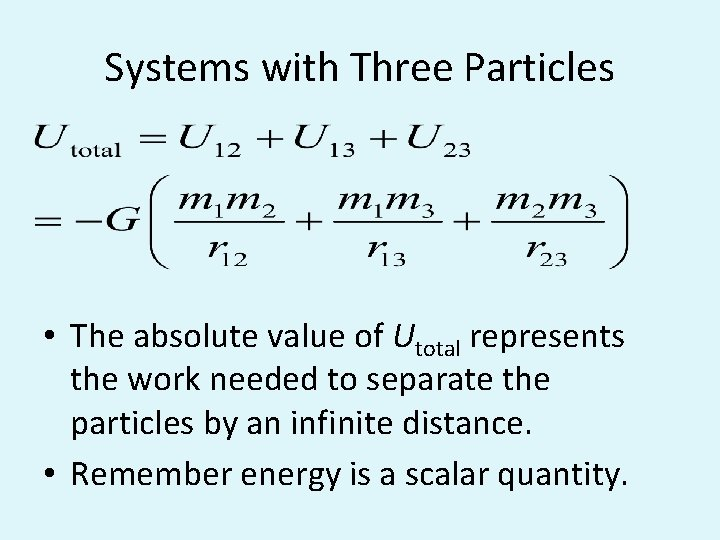Systems with Three Particles • The absolute value of Utotal represents the work needed to separate the particles by an infinite distance. • Remember energy is a scalar quantity.Configurations of Masses • Gravitational Forces are added using the vector component method. • To find the Gravitational Potential Energy of the configuration of masses, the individual energies are added as scalars. • A force would have to supply an amount of energy equal to the individual energy in order to separate the masses by an infinite distance.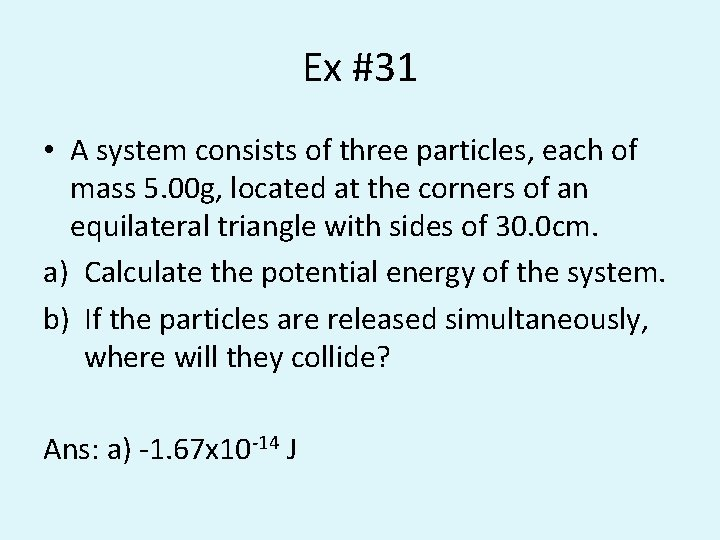Ex #31 • A system consists of three particles, each of mass 5. 00 g, located at the corners of an equilateral triangle with sides of 30. 0 cm. a) Calculate the potential energy of the system. b) If the particles are released simultaneously, where will they collide? Ans: a) -1. 67 x 10 -14 J